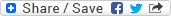# Temperature Scales and ConversionsIR Talk
The Snell Group

Temperature is one of the most frequently measured variables on Earth, but is generally one of the least understood. We discuss temperature measurement scales in Level 1 - Thermographic Applications. However, Level 2 - Advanced Infrared Thermography is where we talk the most about temperature and the necessity of the putting temperature into context for the end user of your thermographic report.

As part of our discussion of temperatures, we discuss the relationship between Fahrenheit, Celsius, and how to convert from one scale to another. The formula for converting Fahrenheit to Celsius is (F-32) ÷ 1.8. When converting from Celsius to Fahrenheit the formula is (C X 1.8) +32. Also, we discuss the conversion of temperature difference from one scale to the other using the value of 1.8 as a multiplier or divisor.

Fahrenheit and Celsius are both referred to as “relative” temperature scales because they are based on relative energy values. Both Fahrenheit and Celsius use the freezing and boiling points of water as reference points for the rest of the respective scales, hence, the term “relative”. Here’s an important point to remember: Fahrenheit and Celsius both reference the same two identical physical levels of heat energy - freezing and boiling of water. However, they assign to vastly different numerical values to points on those scales. Between freezing and boiling in Fahrenheit we have 180 divisions. From 32°F to 212°F is 180. Then between those same two physical points in Celsius we have 100 divisions. 0°C to 100°C is 100 divisions. Do you understand the concepts? Therefore, when you compare the two scales, there’s a 1.8 to 1.0 ratio between Fahrenheit and Celsius. This is where the 1.8 values in the conversion tables and the differential conversion come from.

People tend to get confused though when converting from one scale to another. When converting actual temperatures from one scale to the other, the full conversion formula should be used. When converting differences between two objects from one scale to the other, we either multiply or divide by 1.8 depending on whether we’re going from Celsius to Fahrenheit or the other way. This is the point that confuses some people. I will break it down a bit.

Take a temperature figure, say 80°F. When I assign the value of 80°F to a surface, or air, or whatever, I’m saying the magnitude of heat energy is equivalent to 80°F. When converting a magnitude of energy from one scale to another, I would use the full length conversion formula.  Let’s say I’m inspecting an object, and I compare its temperature to that of a nearby similar object. The difference between them measures 80°F.  In this instance, 80°F is the difference in the temperature measurement between the surfaces, not a magnitude of energy equivalent to 80°F. The temperature differential is 80°F, which might mean one object measures 40°F and the other measures 120°F. Or one might measure 120°F and the other one 200°F. I’m measuring a difference in temperature, not a level of energy present as I would be if I assigned a temperature of 80°F to the object. That’s where the difference is, and why there are different conversion formulas for each circumstance.

Remember, the difference between a magnitude of heat energy and the comparison from one surface to another. When we’re dealing with magnitudes of energy, convert using the full formula. When dealing with differentials, use the 1.8 value multiplier or divisor. It’s easy to get confused, but if you remember these key differences you can avoid making this common mistake in the field.

If you liked this post, you might enjoy our Knowledge Briefs Newsletter. Receive new posts delivered right to your inbox every week!